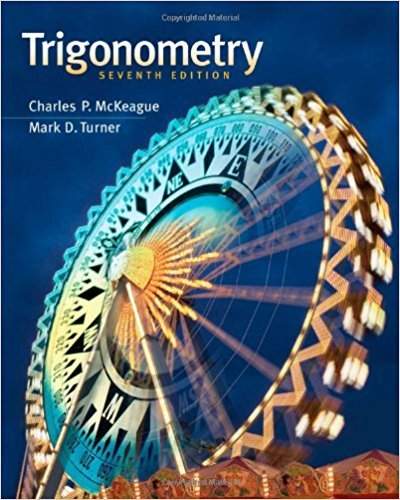×
×

# Solutions for Chapter 4: Graphing and Inverse Functions## Full solutions for Trigonometry | 7th Edition

ISBN: 9781111826857Solutions for Chapter 4: Graphing and Inverse Functions

Solutions for Chapter 4
4 5 0 428 Reviews
27
1
##### ISBN: 9781111826857

This expansive textbook survival guide covers the following chapters and their solutions. Chapter 4: Graphing and Inverse Functions includes 30 full step-by-step solutions. This textbook survival guide was created for the textbook: Trigonometry, edition: 7. Since 30 problems in chapter 4: Graphing and Inverse Functions have been answered, more than 24936 students have viewed full step-by-step solutions from this chapter. Trigonometry was written by and is associated to the ISBN: 9781111826857.

Key Math Terms and definitions covered in this textbook
• Associative Law (AB)C = A(BC).

Parentheses can be removed to leave ABC.

• Back substitution.

Upper triangular systems are solved in reverse order Xn to Xl.

• Cayley-Hamilton Theorem.

peA) = det(A - AI) has peA) = zero matrix.

• Cyclic shift

S. Permutation with S21 = 1, S32 = 1, ... , finally SIn = 1. Its eigenvalues are the nth roots e2lrik/n of 1; eigenvectors are columns of the Fourier matrix F.

• Elimination matrix = Elementary matrix Eij.

The identity matrix with an extra -eij in the i, j entry (i #- j). Then Eij A subtracts eij times row j of A from row i.

• Ellipse (or ellipsoid) x T Ax = 1.

A must be positive definite; the axes of the ellipse are eigenvectors of A, with lengths 1/.JI. (For IIx II = 1 the vectors y = Ax lie on the ellipse IIA-1 yll2 = Y T(AAT)-1 Y = 1 displayed by eigshow; axis lengths ad

• Hessenberg matrix H.

Triangular matrix with one extra nonzero adjacent diagonal.

• Inverse matrix A-I.

Square matrix with A-I A = I and AA-l = I. No inverse if det A = 0 and rank(A) < n and Ax = 0 for a nonzero vector x. The inverses of AB and AT are B-1 A-I and (A-I)T. Cofactor formula (A-l)ij = Cji! detA.

• Kronecker product (tensor product) A ® B.

Blocks aij B, eigenvalues Ap(A)Aq(B).

• Linear combination cv + d w or L C jV j.

• Linear transformation T.

Each vector V in the input space transforms to T (v) in the output space, and linearity requires T(cv + dw) = c T(v) + d T(w). Examples: Matrix multiplication A v, differentiation and integration in function space.

• Lucas numbers

Ln = 2,J, 3, 4, ... satisfy Ln = L n- l +Ln- 2 = A1 +A~, with AI, A2 = (1 ± -/5)/2 from the Fibonacci matrix U~]' Compare Lo = 2 with Fo = O.

• Minimal polynomial of A.

The lowest degree polynomial with meA) = zero matrix. This is peA) = det(A - AI) if no eigenvalues are repeated; always meA) divides peA).

• Normal matrix.

If N NT = NT N, then N has orthonormal (complex) eigenvectors.

• Pivot.

The diagonal entry (first nonzero) at the time when a row is used in elimination.

• Rank one matrix A = uvT f=. O.

Column and row spaces = lines cu and cv.

• Symmetric factorizations A = LDLT and A = QAQT.

Signs in A = signs in D.

• Transpose matrix AT.

Entries AL = Ajj. AT is n by In, AT A is square, symmetric, positive semidefinite. The transposes of AB and A-I are BT AT and (AT)-I.

• Vector v in Rn.

Sequence of n real numbers v = (VI, ... , Vn) = point in Rn.

• Wavelets Wjk(t).

Stretch and shift the time axis to create Wjk(t) = woo(2j t - k).

×